# 第7周

## 十二、支持向量机(Support Vector Machines)

### 12.1 优化目标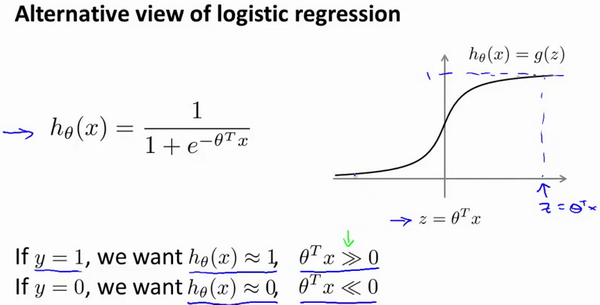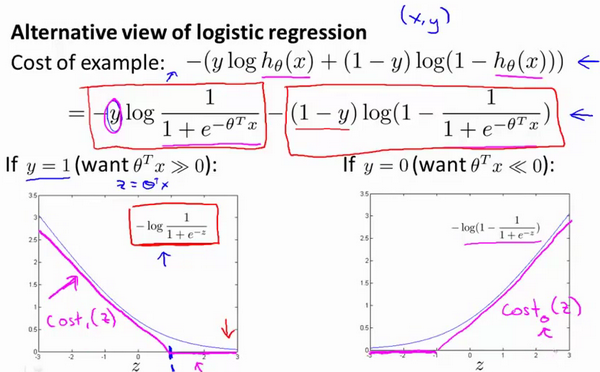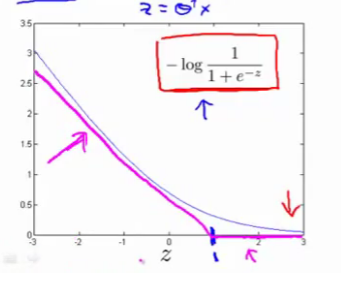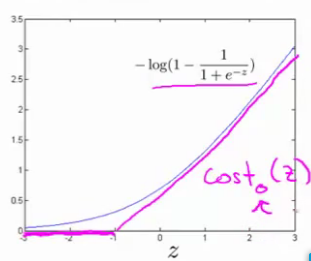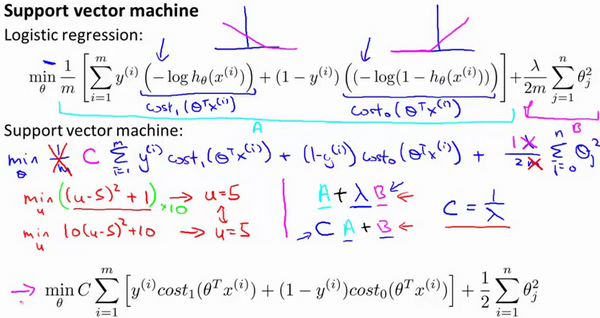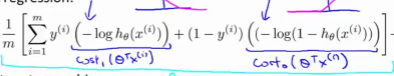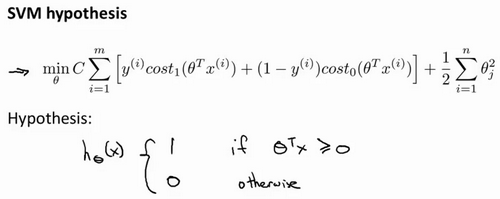### 12.2 大边界的直观理解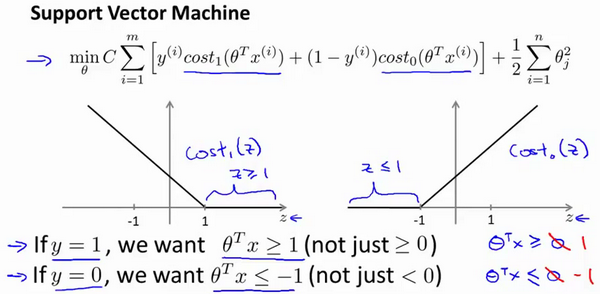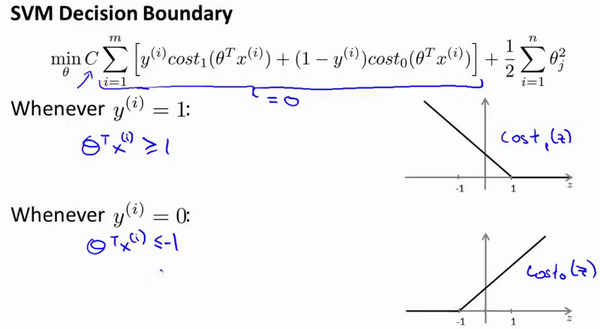$\min_\limits{\theta}C\sum_\limits{i=1}^{m}\left[y^{(i)}{\cos}t_{1}\left(\theta^{T}x^{(i)}\right)+\left(1-y^{(i)}\right){\cos}t\left(\theta^{T}x^{(i)}\right)\right]+\frac{1}{2}\sum_\limits{i=1}^{n}\theta^{2}_{j}$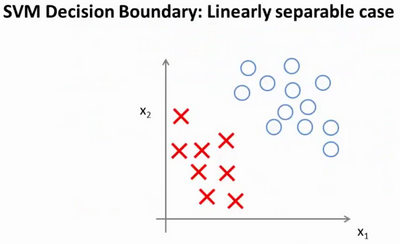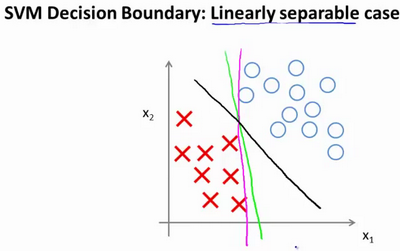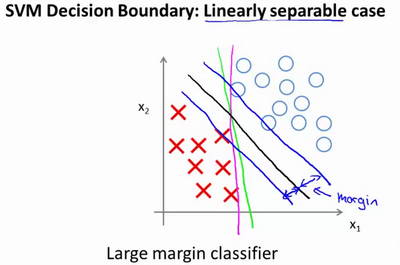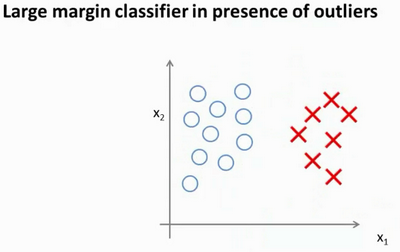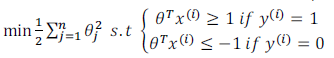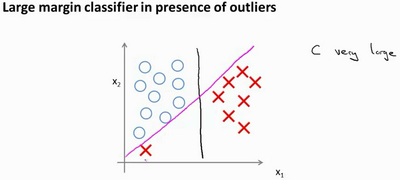$C$ 较大时，相当于 $\lambda$ 较小，可能会导致过拟合，高方差。

$C$ 较小时，相当于$\lambda$较大，可能会导致低拟合，高偏差。

### 12.3 大边界分类背后的数学（选修）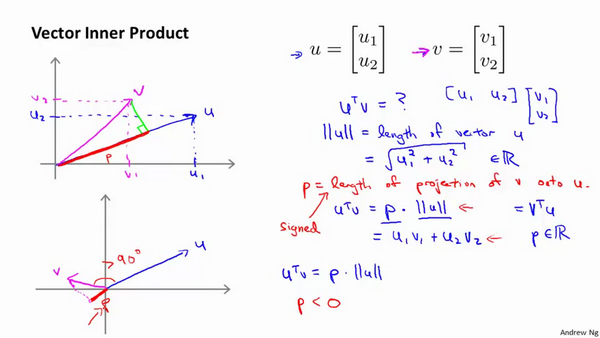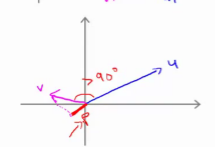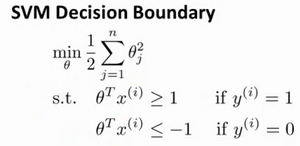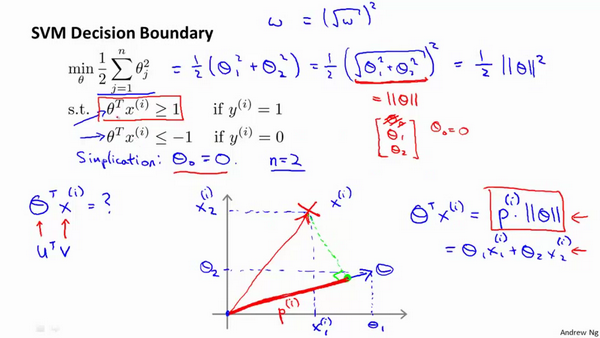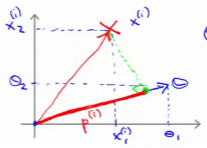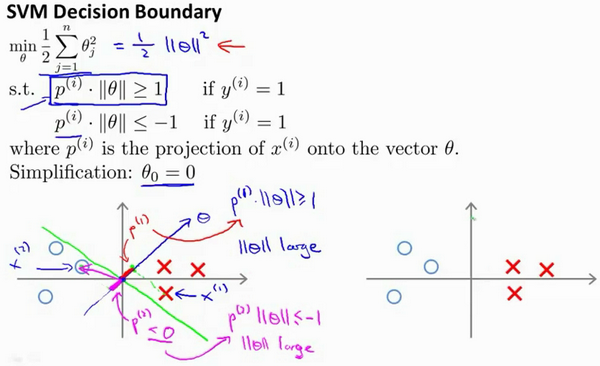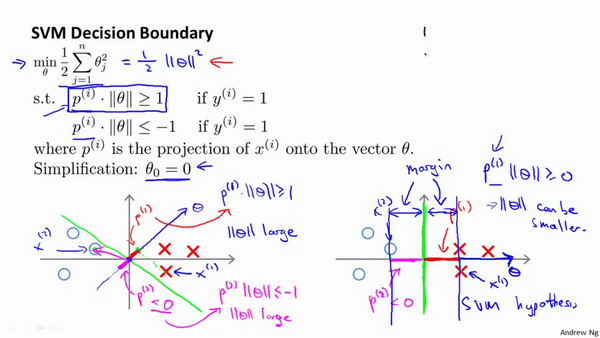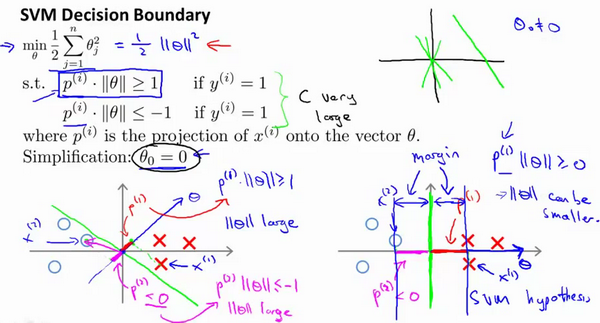### 12.4 核函数1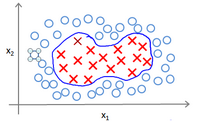...得到$h_θ(x)={{\theta }_{1}}f_1+{{\theta }_{2}}f_2+...+{{\theta }_{n}}f_n$。然而，除了对原有的特征进行组合以外，有没有更好的方法来构造$f_1,f_2,f_3$？我们可以利用核函数来计算出新的特征。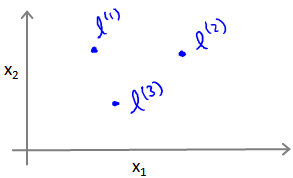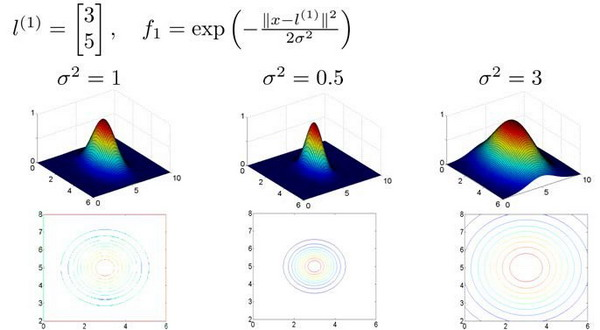### 12.5 核函数2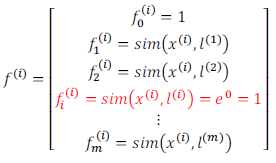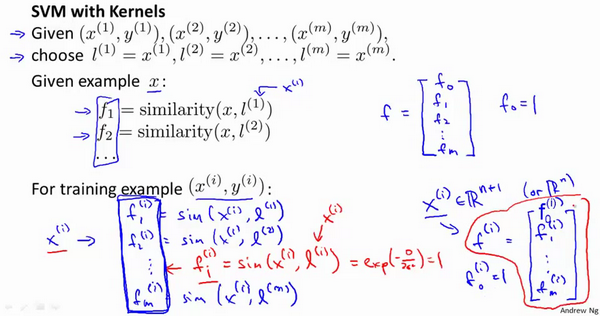• 给定$x$，计算新特征$f$，当$θ^Tf>=0$ 时，预测 $y=1$，否则反之。

$min C\sum\limits_{i=1}^{m}{[{{y}^{(i)}}cos {{t}_{1}}}( {{\theta }^{T}}{{f}^{(i)}})+(1-{{y}^{(i)}})cos {{t}_{0}}( {{\theta }^{T}}{{f}^{(i)}})]+\frac{1}{2}\sum\limits_{j=1}^{n=m}{\theta _{j}^{2}}$ 在具体实施过程中，我们还需要对最后的正则化项进行些微调整，在计算$\sum{_{j=1}^{n=m}}\theta _{j}^{2}={{\theta}^{T}}\theta$时，我们用$θ^TMθ$代替$θ^Tθ$，其中$M$是根据我们选择的核函数而不同的一个矩阵。这样做的原因是为了简化计算。

$C=1/\lambda$

$C$ 较大时，相当于$\lambda$较小，可能会导致过拟合，高方差；

$C$ 较小时，相当于$\lambda$较大，可能会导致低拟合，高偏差；

$\sigma$较大时，可能会导致低方差，高偏差；

$\sigma$较小时，可能会导致低偏差，高方差。

### 12.6 使用支持向量机

1、是提出参数$C$的选择。我们在之前的视频中讨论过误差/方差在这方面的性质。

2、你也需要选择内核参数或你想要使用的相似函数，其中一个选择是：我们选择不需要任何内核参数，没有内核参数的理念，也叫线性核函数。因此，如果有人说他使用了线性核的SVM（支持向量机），这就意味这他使用了不带有核函数的SVM（支持向量机）。

$n$为特征数，$m$为训练样本数。

(1)如果相较于$m$而言，$n$要大许多，即训练集数据量不够支持我们训练一个复杂的非线性模型，我们选用逻辑回归模型或者不带核函数的支持向量机。

(2)如果$n$较小，而且$m$大小中等，例如$n$在 1-1000 之间，而$m$在10-10000之间，使用高斯核函数的支持向量机。

(3)如果$n$较小，而$m$较大，例如$n$在1-1000之间，而$m$大于50000，则使用支持向量机会非常慢，解决方案是创造、增加更多的特征，然后使用逻辑回归或不带核函数的支持向量机。